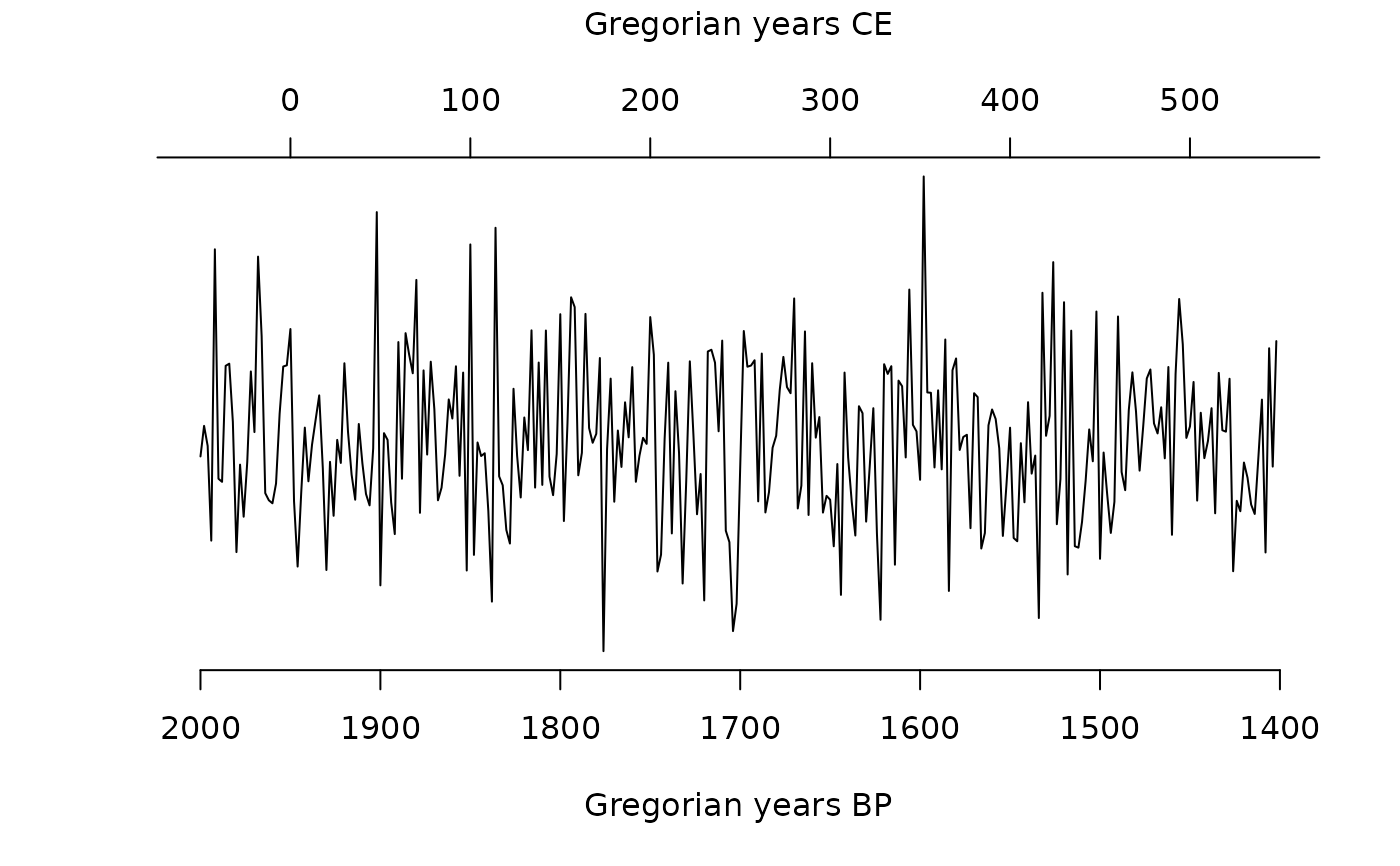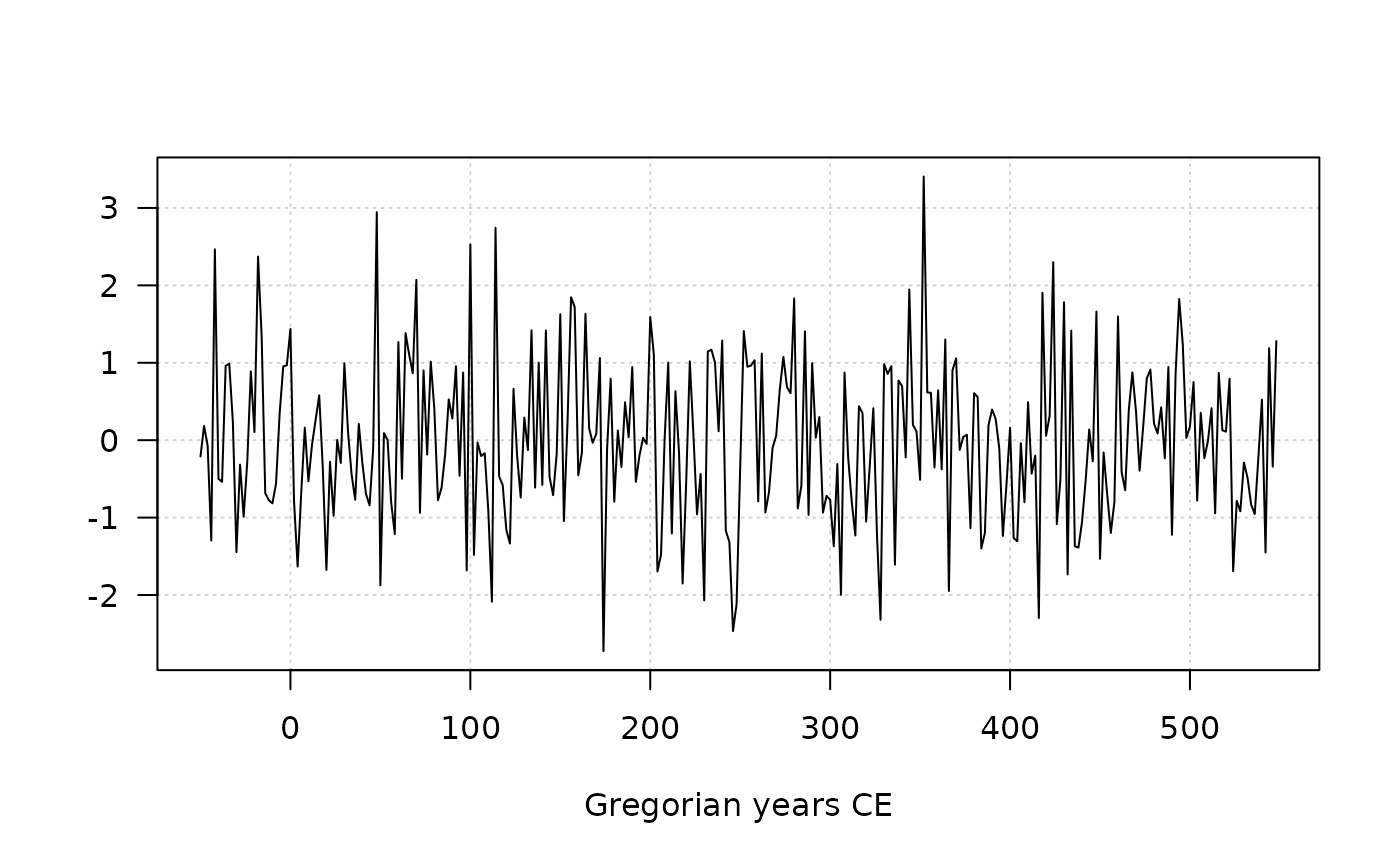Time Series Plotting Functions

## Usage

year_axis(
side,
at = NULL,
format = c("a", "ka", "Ma", "Ga"),
labels = TRUE,
calendar = getOption("aion.last_calendar"),
current_calendar = getOption("aion.last_calendar"),
...
)

## Arguments

side

An integer specifying which side of the plot the axis is to be drawn on. The axis is placed as follows: 1=below, 2=left, 3=above and 4=right.

at

A numeric vector giving the points at which tick-marks are to be drawn. If NULL, tickmark locations are computed.

format

A character string specifying the prefix. It should be one of "a", "ka", "Ma" or "Ga". If TRUE, a good guess for an appropriate format is made.

labels

A logical scalar specifying whether annotations are to be made at the tickmarks, or a vector of character strings to be placed at the tickpoints.

calendar

A TimeScale object specifying the target calendar (see calendar()).

current_calendar

A TimeScale object specifying the calendar used by the last call to plot().

...

Further parameters to be passed to graphics::axis(). (e.g. graphical parameters).

## Value

year_axis() is called it for its side-effects.

Other plotting tools: image(), plot()

N. Frerebeau

## Examples

## Create a time-series of 300 observations
## Sampled every two years starting from 2000 BP
X <- series(
object = rnorm(300),
time = seq(2000, by = -2, length.out = 300),
calendar = BP()
)

## Axis
plot(X, axes = FALSE, calendar = BP()) # Remove axes
year_axis(side = 1) # Same calendar as last plot
year_axis(side = 3, calendar = CE()) # Specific calendar
mtext(format(CE()), side = 3, line = 3)## Grid
plot(X, panel.first = graphics::grid())